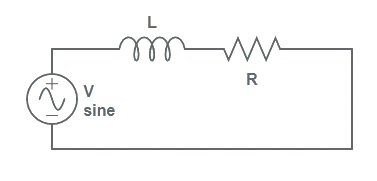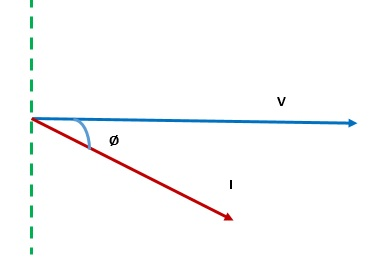# What is Power Factor? – Definition and Formula

Power factor is an important parameter for the calculation of active and reactive power in electrical circuit. It has significance only for AC circuit. For DC circuit, it is not considered for power calculation.Its value is unity for DC circuit and may vary in between zero to one for AC circuit.

We know that power consumed in a DC circuit is given by the multiplication of voltage (V) and current (I). This mean,

Power = VI

But for AC circuit, the above formula is not correct. A third parameter, called the power factor is also included. Thus for AC circuit,

Power = VI x (pf), where pf is power factor of the load.

In this post we will discuss,

### Power Factor Definition:

Power factor is defined as the cosine of angle between the voltage phasor and current phasor in an AC circuit. It is denoted as pf. For an AC circuit, 0≤pf≤1 whereas for DC circuit power factor is always 1.

Let us consider a simple AC circuit to better understand the concept.Since the above circuit is an inductive circuit, the current through the circuit will lag the voltage by some angle. Let us assume, this angle to be Ø. Now we draw the phasor diagram.If Ø is the angle between the voltage and current phasor, therefore as per the definition

pf = CosØ  ………(1)

Since -1≤CosØ≤ 1, therefore the value of pf is always less than or equal to 1 but greater or equal to zero. It cannot be negative as current will either lag or lead the voltage phasor to a maximum of 90º. Therefore magnitude of Ø cannot be more than 90º.

### Formula of Power Factor:

The power factor formulas are listed below:

• If we know the value of angle of lead or lag i.e. Ø then pf can be calculated using information (1).

pf = CosØ

• It can also be calculated from the power triangle. Since active power = VICosØ and apparent power = VI, it can be calculated by calculating the ratio of Active Power and Apparent Power. The formula for pf in terms of active and apparent power is given as

pf = Active Power / Apparent Power

### Power Factor for Resistance, Capacitor and Inductor:

• The current flowing through the resistance is always in phase with the applied voltage. This means that the angle between them is 0º and hence

pf = Cos0 = 1

This means that a resistance will only consume active power. It will not consume any reactive power.

• Current through the inductor lags the applied voltage by 90º. Therefore the angle between them is 90 degree and hence

pf = Cos90 = 0

Thus an inductor will not consume any active power. It only consumes reactive power.

• Similarly, current through capacitor leads the voltage by 90º. Therefore

pf = Cos90 = 0

A capacitor is thus generator of reactive power. It does not consume any active power.

### Power Factor Calculator:

You can download the pf calculator from the link below. This pf calculator, calculates the pf for a load, correction capacitor and kVA rating of Synchronous condenser required for power factor improvement to any any desired value. It is very easy tool, you just need to input some parameter like Active power, frequency, voltage etc and the calculator will calculate everything you need to know.